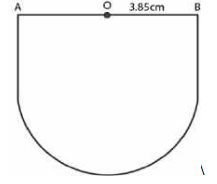# ML Aggarwal Solutions Class 9 Mathematics Solutions for Mensuration Exercise 16.3 in Chapter 16 - Mensuration

Find the perimeter of a semicircular plate of radius 3.85 cm.

It is given that

Radius of semicircular plate = 3.85 cmWe know that

Length of semicircular plat = πr

Perimeter = πr + 2r = r (π + 2)

Substituting the values

= 3.85 (22/7 + 2)

By further calculation

= 3.85 × 36/7

= 0.55 × 36

= 19.8 cm

Related Questions

Lido

Courses

Teachers

Book a Demo with us

Syllabus

Maths
CBSE
Maths
ICSE
Science
CBSE

Science
ICSE
English
CBSE
English
ICSE
Coding

Terms & Policies

Selina Question Bank

Maths
Physics
Biology

Allied Question Bank

Chemistry
Connect with us on social media!